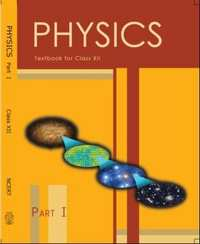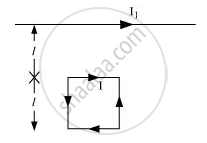Share

# NCERT solutions for Class 12 Physics Textbook chapter 4 - Moving Charges and Magnetism [Latest edition]

Textbook page

#### Chapters## Chapter 4: Moving Charges and Magnetism

#### NCERT solutions for Class 12 Physics Textbook Chapter 4 Moving Charges and Magnetism [Pages 169 - 170]

Q 1 | Page 169

A circular coil of wire consisting of 100 turns, each of radius 8.0 cm carries a current of 0.40 A. What is the magnitude of the magnetic field at the centre of the coil?

Q 2 | Page 169

A long straight wire carries a current of 35 A. What is the magnitude of the field at a point 20 cm from the wire?

Q 3 | Page 169

A long straight wire in the horizontal plane carries a current of 50 A in north to south direction. Give the magnitude and direction of at a point 2.5 m east of the wire.

Q 4 | Page 169

A horizontal overhead power line carries a current of 90 A in east to west direction. What is the magnitude and direction of the magnetic field due to the current 1.5 m below the line?

Q 5 | Page 169

What is the magnitude of magnetic force per unit length on a wire carrying a current of 8 A and making an angle of 30º with the direction of a uniform magnetic field of 0.15 T?

Q 6 | Page 169

A 3.0 cm wire carrying a current of 10 A is placed inside a solenoid perpendicular to its axis. The magnetic field inside the solenoid is given to be 0.27 T. What is the magnetic force on the wire?

Q 7 | Page 169

Two long and parallel straight wires A and B carrying currents of 8.0 A and 5.0 A in the same direction are separated by a distance of 4.0 cm. Estimate the force on a 10 cm section of wire A.

Q 8 | Page 169

A closely wound solenoid 80 cm long has 5 layers of windings of 400 turns each. The diameter of the solenoid is 1.8 cm. If the current carried is 8.0 A, estimate the magnitude of inside the solenoid near its centre.

Q 9 | Page 169

A square coil of side 10 cm consists of 20 turns and carries a current of 12 A. The coil is suspended vertically and the normal to the plane of the coil makes an angle of 30º with the direction of a uniform horizontal magnetic field of magnitude 0.80 T. What is the magnitude of torque experienced by the coil?

Q 10 | Page 169

Two moving coil meters, M1 and M2 have the following particulars:

R1 = 10 Ω, N1 = 30,

A1 = 3.6 × 10–3 m2B1 = 0.25 T

R2 = 14 Ω, N2 = 42,

A2 = 1.8 × 10–3 m2B2 = 0.50 T

(The spring constants are identical for the two meters).

Determine the ratio of (a) current sensitivity and (b) voltage sensitivity of M2 and M1.

Q 11 | Page 169

In a chamber, a uniform magnetic field of 6.5 G (1 G = 10–4 T) is maintained. An electron is shot into the field with a speed of 4.8 × 106 m s–1 normal to the field. Explain why the path of the electron is a circle. Determine the radius of the circular orbit. (= 1.6 × 10–19 C, me= 9.1×10–31 kg)

Q 12 | Page 169

In Exercise 4.11 obtain the frequency of revolution of the electron in its circular orbit. Does the answer depend on the speed of the electron? Explain.

Q 13 | Page 169

a) A circular coil of 30 turns and radius 8.0 cm carrying a current of 6.0 A is suspended vertically in a uniform horizontal magnetic field of magnitude 1.0 T. The field lines make an angle of 60º with the normal of the coil. Calculate the magnitude of the counter torque that must be applied to prevent the coil from turning.

(b) Would your answer change, if the circular coil in (a) were replaced by a planar coil of some irregular shape that encloses the same area? (All other particulars are also unaltered.)

Q 14 | Page 170

Two concentric circular coils X and Y of radii 16 cm and 10 cm, respectively, lie in the same vertical plane containing the north to south direction. Coil X has 20 turns and carries a current of 16 A; coil Y has 25 turns and carries a current of 18 A. The sense of the current in X is anticlockwise, and clockwise in Y, for an observer looking at the coils facing west. Give the magnitude and direction of the net magnetic field due to the coils at their centre.

Q 15 | Page 170

A magnetic field of 100 G (1 G = 10−4 T) is required which is uniform in a region of linear dimension about 10 cm and area of cross-section about 10−3 m2. The maximum current-carrying capacity of a given coil of wire is 15 A and the number of turns per unit length that can be wound round a core is at most 1000 turns m−1. Suggest some appropriate design particulars of a solenoid for the required purpose. Assume the core is not ferromagnetic

#### NCERT solutions for Class 12 Physics Textbook Chapter 4 Moving Charges and Magnetism Extra questions

Write the expression for the magnetic moment vecmdue to a planar square loop of side ‘l’ carrying a steady current I in a vector form.

In the given figure this loop is placed in a horizontal plane near a long straight conductor carrying a steady current I1 at a distance l as shown. Give reason to explain that the loop will experience a net force but no torque. Write the expression for this force acting on the loop.## Chapter 4: Moving Charges and Magnetism## NCERT solutions for Class 12 Physics Textbook chapter 4 - Moving Charges and Magnetism

NCERT solutions for Class 12 Physics Textbook chapter 4 (Moving Charges and Magnetism) include all questions with solution and detail explanation. This will clear students doubts about any question and improve application skills while preparing for board exams. The detailed, step-by-step solutions will help you understand the concepts better and clear your confusions, if any. Shaalaa.com has the CBSE Class 12 Physics Textbook solutions in a manner that help students grasp basic concepts better and faster.

Further, we at Shaalaa.com provide such solutions so that students can prepare for written exams. NCERT textbook solutions can be a core help for self-study and acts as a perfect self-help guidance for students.

Concepts covered in Class 12 Physics Textbook chapter 4 Moving Charges and Magnetism are The Magnetic Dipole Moment of a Revolving Electron, Circular Current Loop as a Magnetic Dipole, Torque on a Rectangular Current Loop in a Uniform Magnetic Field, Magnetic Field on the Axis of a Circular Current Loop, Motion in a Magnetic Field, Velocity Selector, Solenoid and the Toroid - the Toroid, Solenoid and the Toroid - the Solenoid, Magnetic Diapole, Moving Coil Galvanometer, Torque on a Current Loop in Magnetic Field, Force Between Two Parallel Currents, the Ampere, Force on a Current - Carrying Conductor in a Uniform Magnetic Field, Cyclotron, Force on a Moving Charge in Uniform Magnetic and Electric Fields, Straight and Toroidal Solenoids (Only Qualitative Treatment), Ampere’s Circuital Law, Magnetic Field Due to a Current Element, Biot-Savart Law, Oersted’s Experiment, Magnetic Force.

Using NCERT Class 12 solutions Moving Charges and Magnetism exercise by students are an easy way to prepare for the exams, as they involve solutions arranged chapter-wise also page wise. The questions involved in NCERT Solutions are important questions that can be asked in the final exam. Maximum students of CBSE Class 12 prefer NCERT Textbook Solutions to score more in exam.

Get the free view of chapter 4 Moving Charges and Magnetism Class 12 extra questions for Class 12 Physics Textbook and can use Shaalaa.com to keep it handy for your exam preparation

S